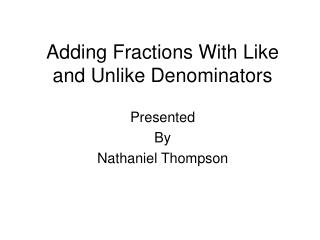DownloadDownload PresentationAdding Fractions With Like and Unlike Denominators

Adding Fractions With Like and Unlike Denominators

Télécharger la présentationAdding Fractions With Like and Unlike Denominators

- - - - - - - - - - - - - - - - - - - - - - - - - - - E N D - - - - - - - - - - - - - - - - - - - - - - - - - - -
Presentation Transcript

1. Adding Fractions With Like and Unlike Denominators Presented By Nathaniel Thompson

2. What is a fraction? A fraction is a representation of a part of a whole unit. A fraction has two parts, a numerator and a denominator.

3. 2 3 The top number (2) is called the numerator. The bottom number (3) is called the denominator. This fraction represent two thirds of the whole unit.

4. 7 + 6 15 15 When adding fractions with denominators that are the same, simply add the two numerators. The denominator remains the same. Adding Fractions With Like Denominators

5. 7 + 6 = 15 15 13 15

6. 1 + 1 8 4 Before fractions with different denominators can be added, the denominators must be changed so that they are the same. You must find a common denominator. Adding Fractions With Unlike Denominators

7. 1 + 1 8 4 To find the least common denominator, find the least common multiple of 4 and 8 4: 4, 8… 8: 8… The least common denominator of 4 and 8 is 8. Adding Fractions With Unlike Denominators

8. 1 + 1 8 4 Since the denominator of the first fraction is 8, the first fraction remains the same. The denominator of the second fraction (4) must be changed to 8. Adding Fractions With Unlike Denominators

9. 1 + 1x2 8 4x2 Because the denominator (4) is multiplied by 2, the numerator (1) must also be multiplied by 2. Adding Fractions With Unlike Denominators

10. 1 + 2 8 8 The New Addition Problem Becomes Adding Fractions With Unlike Denominators

11. 1 + 2 = 8 8 3 8 Adding Fractions With Unlike Denominators

12. The End